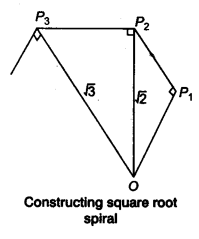NCERT Solutions for Class 9 Maths Chapter 1 Number Systems Ex 1.2 are part of NCERT Solutions for Class 9 Maths. Here we have given NCERT Solutions for Class 9 Maths Chapter 1 Number Systems Ex 1.2.

 Board CBSE Textbook NCERT Class Class 9 Subject Maths Chapter Chapter 1 Chapter Name Number Systems Exercise Ex 1.2 Number of Questions Solved 4 Category NCERT Solutions

## NCERT Solutions for Class 9 Maths Chapter 1 Number Systems Ex 1.2

Question 1.
State whether the following statements are true or false. Justify your answers.
(i) Every irrational number is a real number.
(ii) Every point on the number line is of the form √m , where m is a natural number.
(iii) Every real number is an irrational number.
Solution:
(i) True (∵ Real numbers = Rational numbers + Irrational numbers.)
(ii) False (∵ no negative number can be the square root of any natural number.)
(iii) False (∵ rational numbers are also present in the set of real numbers.)

Question 2.
Are the square roots of all positive integers irrational? If not, give an example of the square root of a number that is a rational number.
Solution:
No, the square roots of all positive integers are not irrational.
e.g., √l6 = 4
Here, ‘4’ is a rational number.

Question 3.
Show how √5 can be represented on the number line.
Solution:Now, take O as centre OP = √5 as radius, draw an arc, which intersects the line at point R. .
Hence, the point R represents √5.

Question 4.
Classroom activity (constructing the ‘square root spiral’).
Solution:
Take a large sheet of paper and construct the ‘square root spiral’ in the following fashion. Start with a point O and draw a line segment OP1, of unit lengths Draw a line segment P1, P2 perpendicular to OP1 of unit length (see figure).Now, draw a line segment P2P3 perpendicular to OP2. Then draw a line segment P3P4 perpendicular to OP3. Continuing in this manner, you can get the line segment Pn-1 Pn by drawing a line segment of unit length perpendicular to
OPn-1. In this manner, you will have created the points P2, P3,…… Pn,….. and
joined them to create a beautiful spiral depicting √2,√3,√4,……

We hope the NCERT Solutions for Class 9 Maths Chapter 1 Number Systems Ex 1.2, help you. If you have any query regarding NCERT Solutions for Class 9 Maths Chapter 1 Number Systems Ex 1.2, drop a comment below and we will get back to you at the earliest.